PSA2RV7 - Classwork---Geometry, M1, Lesson 33 (G.CO.A.1, G.CO.C.9, G.CO.C.10)

1. Fact/Property: Two angles that form a linear pair are supplementary.

Based on the figure below, what is the measure of ∠b? Do not include units in your answer.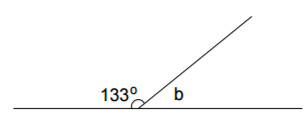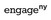Modified from EngageNY ©Great Minds Disclaimer

Type your answer below as a number (example: 5, 3.1, 4 1/2, or 3/2):

2. Fact/Property: The sum of the measures of all adjacent angles formed by three or more rays with the same vertex is 360°.

Based on the figure below, what is the measure of ∠g? Do not include units in your answer.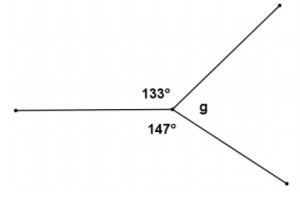Modified from EngageNY ©Great Minds Disclaimer

Type your answer below as a number (example: 5, 3.1, 4 1/2, or 3/2):

3. Fact/Property: Vertical angles have equal measure.

Use the fact that linear pairs form supplementary angles to prove that vertical angles are equal in measure.Modified from EngageNY ©Great Minds Disclaimer

4. Fact/Property: The bisector of an angle is a ray in the interior of the angle such that the two adjacent angles formed by it have equal measure.

In the diagram below,is the bisector of ∠ABD, which measures 64°. What is the measure of ∠ABC? Do not include units in your answer.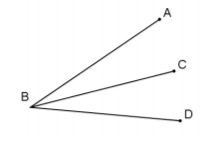Modified from EngageNY ©Great Minds Disclaimer

Type your answer below as a number (example: 5, 3.1, 4 1/2, or 3/2):
Part A)

5. Fact/Property: The perpendicular bisector of a segment is the line that passes through the midpoint of a line segment and is perpendicular to the line segment.

In the diagram below,is the perpendicular bisector of, andis the angle bisector of ∠ACD.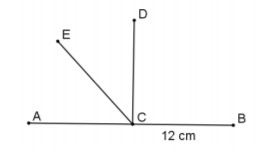a) Find the measure of. Do not include units in your answer.Modified from EngageNY ©Great Minds Disclaimer

Type your answer below as a number (example: 5, 3.1, 4 1/2, or 3/2):
Part B)

b) Find the measures of ∠ECD. Do not include units in your answer.

Type your answer below as a number (example: 5, 3.1, 4 1/2, or 3/2):
Part A)

6. Fact/Property: The sum of the 3 angle measures of any triangle is 180°.

a) Given the labeled figure below, find the measure of ∠DEB. Do not include units in your answer.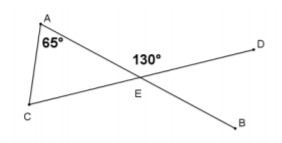Modified from EngageNY ©Great Minds Disclaimer

Type your answer below as a number (example: 5, 3.1, 4 1/2, or 3/2):
Part B)

b) Find the measure of ∠ACE. Do not include units in your answer.

Type your answer below as a number (example: 5, 3.1, 4 1/2, or 3/2):
Part C)

7. Fact/Property: When one angle of a triangle is a right angle, the sum of the measures of the other two angles is 90°.

How is simple arithmetic used to extend the angle sum of a triangle property to justify this property?Modified from EngageNY ©Great Minds Disclaimer

8. Fact/Property: An exterior angle of a triangle is equal to the sum of its two opposite interior angles.

In the diagram below, how is the exterior angle of a triangle property proved?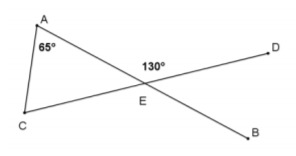Modified from EngageNY ©Great Minds Disclaimer

9. Fact/Property: Base angles of an isosceles triangle are congruent.

The triangle in the figure below is isosceles. How do we know this?Modified from EngageNY ©Great Minds Disclaimer

10. Fact/Property: All angles in an equilateral triangle have equal measure.

Explain how the figure below can be used to demonstrate the equilateral triangle property.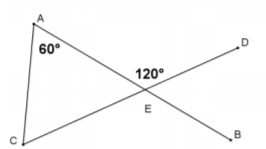Modified from EngageNY ©Great Minds Disclaimer

11. Fact/Property: If a transversal intersects two parallel lines, then the measures of the corresponding angles are equal.

Why does the property specify parallel lines?Modified from EngageNY ©Great Minds Disclaimer

12. Fact/Property: If a transversal intersects two lines such that the measures of the corresponding angles are equal, then the lines are parallel.

The converse of a statement turns the relevant property into an if and only if relationship. Explain how this is related to the guiding question about corresponding angles.Modified from EngageNY ©Great Minds Disclaimer

13. Fact/Property: If a transversal intersects two parallel lines, then the interior angles on the same side of the transversal are supplementary.

This property is proved using (in part) the corresponding angles property. Use the diagram belowto prove that ∠AGH and ∠CHG are supplementary.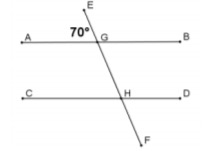Modified from EngageNY ©Great Minds Disclaimer

14. Fact/Property: If a transversal intersects two lines such that the same side interior angles are supplementary, then the lines are parallel.

Given the labeled diagram below, prove that.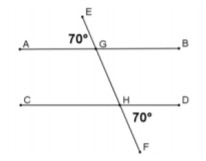Modified from EngageNY ©Great Minds Disclaimer

Part A)

15. Fact/Property: If a transversal intersects two parallel lines, then the measures of alternate interior angles are equal.

a) Name both pairs of alternate interior angles in the diagram below.Modified from EngageNY ©Great Minds Disclaimer

Part B)

b) Based on the figure in Part (a), how many different angle measures are in the diagram?

Type your answer below as a number (example: 5, 3.1, 4 1/2, or 3/2):

16. Fact/Property: If a transversal intersects two lines such that measures of the alternate interior angles are equal, then the lines are parallel.

Although not specifically stated here, the property also applies to alternate exterior angles. Why is this true?Modified from EngageNY ©Great Minds Disclaimer